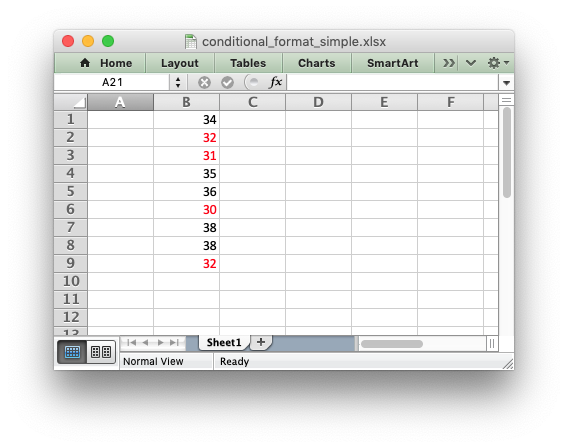libxlsxwriter
conditional_format1.c

A simple example of how to add a conditional format a libxlsxwriter file. Conditional formatting allows you to apply a format to a cell or a range of cells based on certain criteria./*
* An a simple example of how to add conditional formatting to an
* libxlsxwriter file.
*
* See conditional_format.c for a more comprehensive example.
*
* Copyright 2014-2021, John McNamara, jmcnamara@cpan.org
*
*/
#include "xlsxwriter.h"
int main() {
lxw_workbook *workbook = workbook_new("conditional_format_simple.xlsx");
lxw_worksheet *worksheet = workbook_add_worksheet(workbook, NULL);
/* Write some sample data. */
worksheet_write_number(worksheet, CELL("B1"), 34, NULL);
worksheet_write_number(worksheet, CELL("B2"), 32, NULL);
worksheet_write_number(worksheet, CELL("B3"), 31, NULL);
worksheet_write_number(worksheet, CELL("B4"), 35, NULL);
worksheet_write_number(worksheet, CELL("B5"), 36, NULL);
worksheet_write_number(worksheet, CELL("B6"), 30, NULL);
worksheet_write_number(worksheet, CELL("B7"), 38, NULL);
worksheet_write_number(worksheet, CELL("B8"), 38, NULL);
worksheet_write_number(worksheet, CELL("B9"), 32, NULL);
/* Add a format with red text. */
lxw_format *custom_format = workbook_add_format(workbook);
/* Create a conditional format object. A static object would also work. */
lxw_conditional_format *conditional_format = calloc(1, sizeof(lxw_conditional_format));
/* Set the format type: a cell conditional: */
conditional_format->type = LXW_CONDITIONAL_TYPE_CELL;
/* Set the criteria to use: */
/* Set the value to which the criteria will be applied: */
conditional_format->value = 33;
/* Set the format to use if the criteria/value applies: */
conditional_format->format = custom_format;
/* Now apply the format to data range. */
worksheet_conditional_format_range(worksheet, RANGE("B1:B9"), conditional_format);
/* Free the object and close the file. */
free(conditional_format);
return workbook_close(workbook);
}
workbook_close
lxw_error workbook_close(lxw_workbook *workbook)
Close the Workbook object and write the XLSX file.
lxw_conditional_format
Worksheet conditional formatting options.
Definition: worksheet.h:1122
lxw_conditional_format::format
lxw_format * format
Definition: worksheet.h:1158
workbook_new
lxw_workbook * workbook_new(const char *filename)
Create a new workbook object.
lxw_conditional_format::type
uint8_t type
Definition: worksheet.h:1127
lxw_worksheet
Struct to represent an Excel worksheet.
Definition: worksheet.h:2107
lxw_format
Struct to represent the formatting properties of an Excel format.
Definition: format.h:358
RANGE
#define RANGE(range)
Convert an Excel A1:B2 range into a (first_row, first_col, last_row, last_col) sequence.
Definition: utility.h:82
LXW_CONDITIONAL_TYPE_CELL
@ LXW_CONDITIONAL_TYPE_CELL
Definition: worksheet.h:237
lxw_workbook
Struct to represent an Excel workbook.
Definition: workbook.h:280
lxw_conditional_format::criteria
uint8_t criteria
Definition: worksheet.h:1135
LXW_COLOR_RED
@ LXW_COLOR_RED
Definition: format.h:217
lxw_conditional_format::value
double value
Definition: worksheet.h:1139
worksheet_write_number
lxw_error worksheet_write_number(lxw_worksheet *worksheet, lxw_row_t row, lxw_col_t col, double number, lxw_format *format)
Write a number to a worksheet cell.
format_set_font_color
void format_set_font_color(lxw_format *format, lxw_color_t color)
Set the color of the font used in the cell.
LXW_CONDITIONAL_CRITERIA_LESS_THAN
@ LXW_CONDITIONAL_CRITERIA_LESS_THAN
Definition: worksheet.h:317
worksheet_conditional_format_range
lxw_error worksheet_conditional_format_range(lxw_worksheet *worksheet, lxw_row_t first_row, lxw_col_t first_col, lxw_row_t last_row, lxw_col_t last_col, lxw_conditional_format *conditional_format)
Add a conditional format to a worksheet range.
CELL
#define CELL(cell)
Convert an Excel A1 cell string into a (row, col) pair.
Definition: utility.h:45